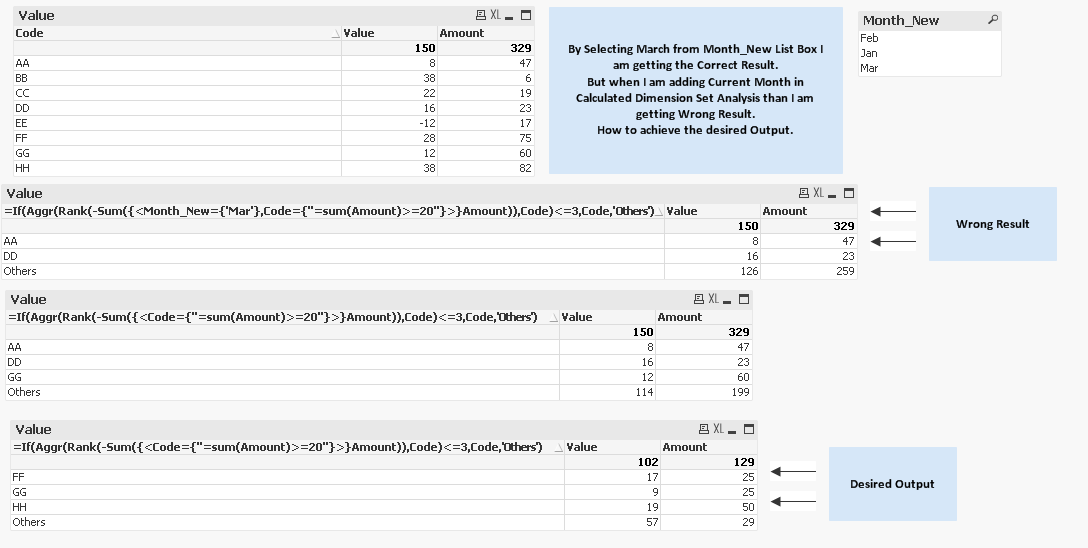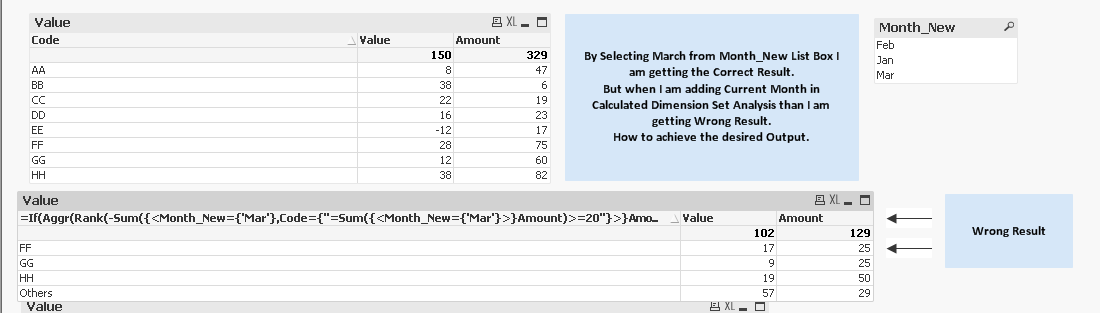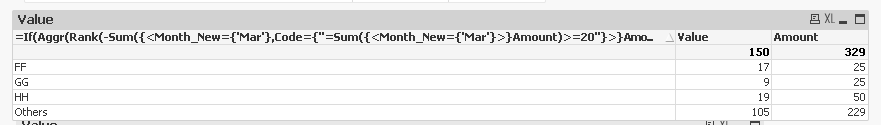Announcements
Product Release Webinar: Qlik Insider airing December 6! REGISTER TODAY!
cancel
Showing results for
Did you mean:Not applicable

## Front end Selection Working and Set Analysis not working

Hi All

By Selecting March from Month_New List Box I am getting the Correct Result.

But when I am adding Current Month in Calculated Dimension Set Analysis than I am

getting Wrong Result.

How to achieve the desired Output.

See this:I have Attached the qvw for Reference.

Regards

Cire

1 Solution

Accepted SolutionsMVP

Here are the changes that you need

Calculated dimension:

=If(Aggr(Rank(-Sum({<Month_New={'Mar'},Code={"=Sum({<Month_New={'Mar'}>}Amount)>=20"}>}Amount)),Code)<=3,Code,'Others')

Expressions

Sum({<Month_New={'Mar'}>} Value)

Sum({<Month_New={'Mar'}>} Amount)11 RepliesNot applicable
Author

Regards

CireMVP

Here are the changes that you need

Calculated dimension:

=If(Aggr(Rank(-Sum({<Month_New={'Mar'},Code={"=Sum({<Month_New={'Mar'}>}Amount)>=20"}>}Amount)),Code)<=3,Code,'Others')

Expressions

Sum({<Month_New={'Mar'}>} Value)

Sum({<Month_New={'Mar'}>} Amount)Not applicable
Author

Sir I can only use the Max Month in Calculated Dimension.

In my Actual Application, the Expressions are so complex.

What to do now??? Any workaround for this.MVP

Use Max Month? Not sure I understand your questionNot applicable
Author

Sir I am saying that I can't use Max Month in my Expressions like this:

Expressions

Sum({<Month_New={'Mar'}>} Value)

Sum({<Month_New={'Mar'}>} Amount)

In my Actual Application, the Expressions are very complex.

I just had shared the sample Application.

Hope you understood my question???

I have to Apply Logic only in the Calculated Dimension.

Calculated dimension:

=If(Aggr(Rank(-Sum({<Code={"=Sum(Amount)>=20"}>}Amount)),Code)<=3,Code,'Others')

Is there a way or any workaround.MVP

I don't think this can be done without changing the expression. You might be able to make use of alternate state to get this done. Is alternate state an option?Not applicable
Author

If we are using Sum(Amount)>=20 in Set Analysis in Calculated Dimension than why can't we use other condition for Max Month in set Analysis.

That's very strange behaviour or is there something Logical here which I am missing.MVP

You bring up a good point, let me take a look at thisMVP

Sir you were right, try this:

=If(Aggr(Rank(-Sum({<Month_New={'Mar'},Code={"=Sum({<Month_New={'Mar'}>}Amount)>=20"}>}Amount)),Code, Month_New)<=3,Code,'Others')

Thank you for pushing me to think it throughCommunity Browser